# Grand canonical inverse design of multicomponent colloidal crystals†

Nathan A. Mahynski*a, Runfang Mao b, Evan Pretti b, Vincent K. Shen a and Jeetain Mittalb
aChemical Sciences Division, National Institute of Standards and Technology, Gaithersburg, Maryland 20899-8320, USA. E-mail: nathan.mahynski@nist.gov; Tel: +1 301-975-6836
bDepartment of Chemical and Biomolecular Engineering, Lehigh University, 111 Research Dr., Bethlehem, Pennsylvania 18015-4791, USA

Received 9th December 2019 , Accepted 18th February 2020

First published on 19th February 2020

Inverse design methods are powerful computational approaches for creating colloidal systems which self-assemble into a target morphology by reverse engineering the Hamiltonian of the system. Despite this, these optimization procedures tend to yield Hamiltonians which are too complex to be experimentally realized. An alternative route to complex structures involves the use of several different components, however, conventional inverse design methods do not explicitly account for the possibility of phase separation into compositionally distinct structures. Here, we present an inverse design scheme for multicomponent colloidal systems by combining active learning with a method to directly compute their ground state phase diagrams. This explicitly accounts for phase separation and can locate stable regions of Hamiltonian parameter space which grid-based surveys are prone to miss. Using this we design low-density, binary structures with Lennard-Jones-like pairwise interactions that are simpler than in the single component case and potentially realizable in an experimental setting. This reinforces the concept that ground states of simple, multicomponent systems might be rich with previously unappreciated diversity, enabling the assembly of non-trivial structures with only few simple components instead of a single complex one.

The direct link between a material's macroscopic properties and its microscopic structure has driven extensive research into the design rules underlying rational bottom-up self-assembly.1–3 Colloids, including nanoparticles, are generally facile building blocks whose shape, charge, and surface functionalization can be relatively easily manipulated to control their behavior.3–14 Thus, in principle, features of a colloidal material such as its optoelectronic or mechanical properties, band gaps, or porosity can be rationally designed; however, the breadth of this design space often necessitates computational techniques to engineer the outcome of an assembly process. The technique of choice is generally “inverse design” wherein a desired target structure is initially chosen, and then interaction parameters of the system are reverse engineered via an optimization procedure, such as one based on relative entropy, to yield the target.15–18 Recent works have shown that this approach is capable of identifying interparticle potentials which stabilize low-density structures, as well as a variety of complex symmetries including Frank-Kasper phases;19–21 unfortunately, the potentials which result from this optimization procedure are often complex, possessing non-trivial features with no obvious route to their experimental realization, though recent work has sought to address this issue by focusing on simpler forms of the interaction potential.22,23

An alternative and possibly simpler way to assemble complex colloidal structures involves the use of several “simple” components rather than a single “complex” one.24–27 By this we mean a set of several different colloidal species each with a relatively simple set of pairwise interactions as opposed to a system with a single species whose self-interaction is very complicated. While multicomponent mixtures can be tuned with conventional inverse design,28 this generally proceeds by optimizing a canonical system with a fixed number of particles at the same composition as the target; however, under experimental conditions this number can fluctuate and is more accurately represented by the grand canonical ensemble. Accurately representing the phase behavior becomes significantly more challenging than in single component systems. Gibbs' phase rule stipulates that, when temperature and pressure are fixed, coexistence may occur between as many different compositionally distinct phases as there are components. In spite of this, it is reasonable to expect that when the interparticle potentials are permitted to be arbitrarily complex, multicomponent systems can be found that yield the desired target and are stable with respect to phase separation. However, as constraints on interparticle potentials are imposed to make the designs more experimentally tractable, this becomes less plausible. As a result, there is a need for an inverse design scheme that can account for the possibility of phase separation in multicomponent mixtures.

Here, we report an inverse design scheme to enable the self-assembly of open, exotic lattices which derive their complexity from diversity rather than through chemically infeasible pair potentials. This scheme relies on a recently developed method to compute ground state phase diagrams for multicomponent colloidal systems,29 which allows the fitness of a desired target structure to be determined for a given Hamiltonian. In turn, this may be optimized using an active learning algorithm to design multicomponent systems with simple potentials that assemble into desirable porous structures. To illustrate this inverse design methodology, here we focus on two-dimensional (2D), binary systems with structures that have potential applications in, e.g., creating size-selective porous membranes; however, the underlying principles are extensible to three-dimensional and more multicomponent systems as well. We demonstrate this approach for several different lattices and show how it enables us to identify systems with simple, isotropic interactions that can be used to assemble non-trivial, open lattices.

## 1 Methods

### 1.1 A simple Hamiltonian

We employ a simple pair potential form similar to Lennard-Jones, for which many structures have recently been discovered29 in the ground state (cf.Fig. 1):30,31
 Ui,j(r) = Uri,j(r) +λi,jUai,j(r), (1)
where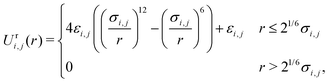(2)
and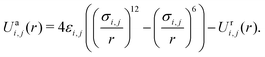(3)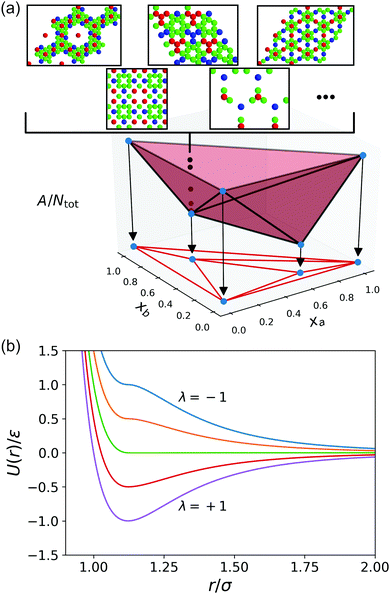Fig. 1 Construction of phase diagrams and a simple Hamiltonian. (a) Example of a ternary phase diagram constructed from the convex hull of specific free energy, A/Ntot, using the lowest energy candidates found at each mole fraction, or stoichiometry, searched. A k-component system yields a k-dimensional hull, and this construction is valid for all multicomponent systems. (b) Depiction of the pair potential (cf.eqn (1)) between species with a chosen λ value which varies smoothly from long-range attractive (λ = 1) to long-range repulsive (λ = −1).

Pairwise interactions are varied by changing the value of λi,j, so that the Hamiltonian can be described by three parameters for a binary system, H = f(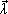) = f(λ1,1, λ1,2, λ2,2). Otherwise, εi,j = σi,j = 1, which were used to non-dimensionalize all energy and length scales, respectively. Interactions were truncated at rcut = 3σ.

### 1.2 Fitness from phase diagrams

Our inverse design scheme is based on the computation of ground state (zero temperature and pressure) phase diagrams for multicomponent colloidal systems.29 These conditions are inspired by those often employed when assembling, e.g., DNA-grafted nanoparticles, where energetically favorable pairwise interactions have characteristic minima well below – kBT (kB is Boltzmann's constant and T is the absolute temperature), and there is no external pressure applied, as in quiescent assembly from a solution phase. In short, an extensive ensemble of different structures is created by using plane symmetry (wallpaper) groups to generate candidates that cover all possible symmetries for all stoichiometries being considered. This involves producing lattices consistent with each group, then solving a constraint satisfaction problem over the lattice to determine all possible structures which have a specified ratio of components. Here, we consider all ratios x:y where max(x,y) ≤ 6. In two dimensions, the result is an extensive set of tessellations that form discrete clusters, strings, as well as regular, uniform (Archimedean), and other tilings [cf.Fig. 1(a)]; for each stoichiometry we considered roughly 106 candidates across all non-trivial wallpaper groups. Once the Hamiltonian, or set of (pairwise) interactions, has been selected the (free) energy of these candidates and the desired target can be relaxed via deterministic minimization and computed; a phase diagram follows from the convex hull of this energy.32 Structures that belong to the hull are stable, whereas at compositions between vertices of the hull, the system will phase separate into the structures whose vertices form the face (or edge) encompassing that composition. At each composition, low energy candidates were compared using a structural similarity metric29 to ensure that each structure on the hull is unique. We have selected a simple Lennard-Jones-like form (cf.eqn (1)) which uses a single parameter, λi,j, to describe how attractive or repulsive each ij-pair interaction is. Thus, a binary mixture's Hamiltonian may be described by= (λ1,1, λ1,2, λ2,2). The system's Gibbs free energy is identical to its potential energy in the ground state. We note that upon incurring additional computational expense to compute, e.g., specific entropies, it is also possible to consider systems at finite temperature and pressure. Each ground state phase diagram required roughly 100 CPU core-hours on a 2.4 GHz Intel Xeon processor to compute, which dominates the total computational requirement of this method.

Using these phase diagrams (cf.Fig. 2) we compute a target's fitness, F, to assess its degree of (meta)stability as a function of parameters in the Hamiltonian. We consider F to be a function of three contributions determined by the phase diagram: (i) the height above the convex hull of (free) energy which defines a structure's degree of (meta)stability, B; (ii) the composition range over which the target is stable, if it falls on the hull, Ω; and (iii) the convexity of the hull, C, at the target's composition. Thus, F = B × Ω × C.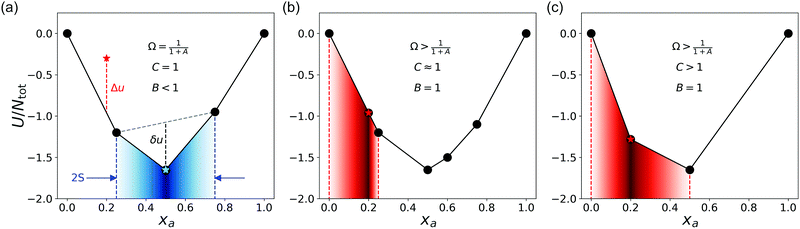Fig. 2 Visual depiction of the optimization process. In (a) the target, whose energy is indicated with a red star at xa = 0.2, is metastable for this set of Hamiltonian parameters. The corresponding values of Ω, C, and B are shown in each panel as the algorithm proceeds; F is the product of all three. Other parameters as described in the main text, S and δu, are illustrated here for a different target (cyan star at xa = 0.5). (b) As the Hamiltonian's parameters are optimized, the target's energy meets the hull, which is itself, a function of the Hamiltonian. (c) Upon further optimization, there are less hull vertices on the hull near the vertex corresponding to the stable target. The remainder of the hull beyond these neighboring vertices does not affect the optimization, though the location and relative depth of the neighbors will depend on the Hamiltonian's parameters.

The first and most important factor, B, we define akin to a Boltzmann factor of the (free) energy difference between the target and the convex hull at the given.

 B = exp(−Δu), (4)
where Δu = (UUhull)/Ntot, Ntot is the total number of particles in the system and U is the structure's potential energy evaluated at[cf.Fig. 2(a)].

The second factor, Ω, weights B by accounting for the range of compositions over which a system will exhibit stable coexistence with the target structure. This is introduced to break “ties” between hulls which all contain the target, and would otherwise all have F = B = 1. A k-component system has a k-dimensional convex hull in (xa,xb,…,xk−1, U/Ntot)-space which provides a phase diagram by projecting into the first (k − 1) dimensions [cf.Fig. 1(a)]. We choose to define this weight as the fraction of the k-component system that should form the target integrated over mole fraction space,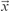.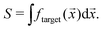(5)

This fraction, ftarget() is simply given by the lever-rule. For example, the shaded blue region in Fig. 2(a) denotes the ftarget() for the cyan target at xa = 0.5; in panels (b) and (c), the shaded red regions denote the results for the red target at xa = 0.2. In the binary case, S is given by the sum of half the differences in mole fraction between that of the target and each of its neighboring vertices on the hull. Ideally, only the target should exist on the hull and solutions with any composition inwill exhibit coexistence with the target structure. In this case, S attains its highest value Smax = 1/k! (cf. ESI):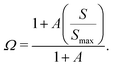(6)

This function is bounded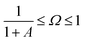, where A ≥ 0. The lower bound occurs when S = 0 and corresponds to the case where the target structure is metastable so it does not belong to the convex hull of (free) energy.32–34 This mathematical form confers the important property that the fitness has a well-defined reference value,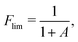(7)
where F < Flim corresponds to a structure that is metastable, while F > Flim corresponds to a stable structure. A is an arbitrary modulus for which we chose A = 3, thus Flim = 0.25. The product 0 < B × Ω ≤ 1 is conveniently bounded; however, it again suffers from the fact that there may exist manywhere a target both belongs to the hull and is only thermodynamically stable over the same range of solution compositions. To break these ties, we introduce the third factor, C, which accounts for the fact that we expect more convex hulls to be more amenable to assembly of the target than shallow ones.
 C = max[δu,0] + 1, (8)
where δu = uuhull′ corresponds to the distance between the target's (free) energy and that of the hull that would be formed if that point were removed from the original hull [cf.Fig. 2(a)].

### 1.3 Molecular dynamics simulations

To validate our designs, molecular dynamics (MD) simulations were performed using LAMMPS35 in the canonical ensemble (NVT) using a Langevin thermostat with LJ reduced units. The total simulation time was at least 1 × 108 steps with a step size of Δτ = 0.05[ε/(2)]−1/2. Larger simulation cells and smaller timesteps yielded the same results. Unless otherwise stated, a total of Ntot = 2000 particles were randomly placed in a square 100σ × 100σ box with periodic boundary conditions, then initially relaxed by energy minimization. When rigid metaparticles (e.g., dimers and tetramers) were used instead of individual particles (monomers), their configurations were determined by performing an initial energy minimization with the pair potential being simulated.

## 2 Inverse design scheme

Now one may optimize the fitness as a function of the parameters in the Hamiltonian (). This amounts to inverse design in a grand canonical sense where we are directly engineering the convex hull of free energy, which determines not only the most stable structure at a fixed composition, but also if it is stable with respect to phase separation into structures with other compositions. We used nonparametric Gaussian process regression (GPR)36 coupled with Bayesian optimization to search for the optimal Hamiltonian. This “active learning” procedure is a powerful global optimization procedure for a diverse set of problems.37,38 GPR constructs a probabilistic model for F() by regressing over all previous observations, {}obs, of different values ofrequiring only that the user specify the form of the covariance function (kernel), k(,′) describing correlations between data points. We used a stationary, anisotropic 5/2 Matérn kernel with automatic relevance determination (cf. ESI).36

The fitness and uncertainty may be calculated at any* desired, after computing the covariance between all pairs of points in the prior, K, between the prior points and a point(s) of interest, K*, and between the point(s) of interest, K**. The predicted fitness of our target structure at* is simply given by: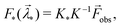(9)
where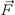obs is the measured fitness of the structure at each {}obs. The variance at this point also follows from the model: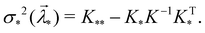(10)

The hyperparameters in the kernel,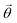, are determined by maximizing the marginal likelihood of the model36 to find the values that lead to the most likely of description of the observations. Active learning seeks to determine the global maximum fitness with a minimum number of new experiments (measured phase diagrams). We employed the regret-free upper confidence bound acquisition function given by:39,40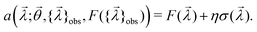(11)

Thus, after fitting the current observations the next query is performed atnext = argmax(a). We set η = 2, thus using the 95% confidence interval to determine this. This cycle of fitting followed by prediction is repeated until a certain computational budget is exhausted or we have reached sufficient convergence, i.e., the optimal fitness value and location does not change significantly over time.

## 3 Results and discussion

### 3.1 Stabilizing an open honeycomb (OHC) lattice

We applied this inverse design procedure to various target structures constrained to have the very simple potential form given by eqn (1) and found this approach is capable of locating stable regions of-space for many non-trivial lattices. For illustration, we first review the approach for the open honeycomb (OHC) lattice in Fig. 3(a–c). We initialized the prior with 25 phase diagrams constructed at each combination of λ1,1 ∈ (−1, 0, +1), λ1,2 ∈ (0, 0.5, 1) and λ2,2 ∈ (−1,0, +1), excluding the= (0, 0, 0) and (−1, 0.5, −1) points (cf. ESI); the former is the trivial case where all interactions are WCA-like, while at the latter this OHC structure has previously been found.29 From the algorithm's perspective, removing it from the prior eliminates all explicit knowledge of where, or even if, the OHC is stable anywhere. This allows us to assess whether or not the algorithm can learn this on its own.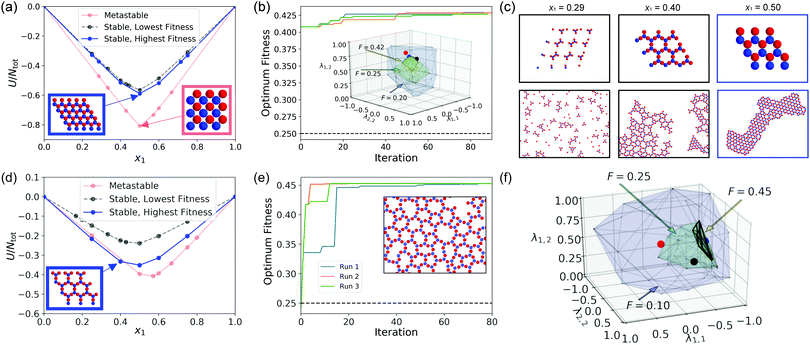Fig. 3 Inverse design of the OHC (a–c) and Kagome ring lattices (d–f). (a) Three representative phase diagrams found during the optimization. The red convex hull corresponds to when the OHC (outlined in blue) is not the lowest energy structure at x1 = 0.5, and so does not belong to the hull (instead a square lattice does). (b) Optimal fitness found over the course of 3 independent runs. In the inset, hulls are drawn around points in-space sampled over the course of optimization that have progressively higher F (Flim = 0.25) as a guide to the eye. The red, black, and blue points correspond to the phase diagrams of the same color in (a). (c) Comparison of structures on the blue phase diagram (top row) to MD simulations at the same mole fractions (bottom row) at T* = 0.02 for the optimal parameters, (λ1,1 = −1.0, λ1,2 ≈ 0.5017, λ2,2 ≈ −0.9325). (d) Representative phase diagrams found during the optimization of the Kagome ring lattice (outlined in blue) at x1 = 0.4 as in (a). (e) Optimal fitness found over the course of 3 independent runs. The inset shows a representative snapshot from MD with the optimal parameters, (λ1,1 = −1.0, λ1,2 ≈ 0.3389, λ2,2 ≈ −0.7211), at T* = 0.02. (f) Hulls are drawn around points observed during these simulations for various fitness thresholds as a guide to the eye. The red, black, and blue points correspond to the phase diagrams of the same color in (d).

The very first point predicted [cf.Fig. 3(b)] was found to have F > 0.4 revealing that regression alone, with a reasonable prior, can be sufficient to locate regions of OHC stability. As the optimization proceeds, it explores regions of higher uncertainty, gradually finding marginally better coordinates until it converges to its optimum= (−1.0, 0.5017, −0.9325) ≈ (−1, 0.5, −1), which turns out to be the missing point from the prior grid. Fig. 3(a) shows representative hulls found during this process. Fig. 3(b) tracks the best fitness found over the course of the optimization procedure; in the inset, convex hulls are drawn around points with increasing F, revealing a structure to the data that shows a single peak around this point, reflecting reasonable behavior of the proposed fitness function. The hulls for the red, black, and blue points correspond to the same colors in Fig. 3(a). The red hull in Fig. 3(a) reveals that an alternating square lattice with x1 = 0.5, rather than the target OHC, is stable at that. Although the OHC does fall on the black hull, there are a number of competitors that manifest closer to x1 = 0.5 than in the optimal hull found (blue).

To test the accuracy of the ground state phase diagrams, MD simulations were performed, some of which are summarized in Fig. 3(c). More detailed results are available in the ESI. The target OHC lattice is stable at the native stoichiometry of the lattice (x1 = 0.5) for the optimal, but as predicted by the phase diagram, loses its stability to a compositionally distinct competitor, namely a Kagome ring, at x1 = 0.4 (and also at x1 = 0.6). Other structures found along the hull are also reflected in the MD simulations, validating the accuracy of the ground state phase diagrams used in grand canonical inverse design.

### 3.2 Stabilizing a low-density Kagome lattice

The OHC lattice has a number of convenient features, namely that it is equimolar (x1 = 0.5) and for this Hamiltonian its fitness has only a single global maximum. However, similar performance has been found for other lattices; for example, we can instead optimize for the Kagome ring41 found at x1 = 0.4 on the optimal OHC phase diagram. Similarly, a single continuous optimal region is found in only a few iterations, though it is located off-center in-space because it is not equimolar [cf.Fig. 3(d–f)]. As in the OHC case, no phase diagram containing this structure was part of the prior. For the Kagome ring, regression alone was not sufficient to immediately predict where this structure would be stable as evidenced by the low fitness value, F < 0.25, for the first iteration in Fig. 3(e). However, this was quickly learned by the algorithm, and a point in the stable region was found on the second iteration of 3 different independent runs. It is important to emphasize that even after the first stable point is found, active learning still uses the uncertainty in the fit of its model to continue exploring other regions of space. Thus, other parts ofspace are visited on subsequent iterations as the model converges and the algorithm is not limited to the initial region where a hull on which the target is stable was initially found.

### 3.3 Application to an Archimedean tile

Both the OHC and Kagome ring lattices show relatively large regions of-space exhibiting stability, and have been previously found via grid-searching.29 Such grid searches are never capable of definitively predicting behavior between discrete points sampled, leaving open the possibility that interesting structures might be stable but only over arbitrarily narrow regions of Hamiltonian parameters between grid points. The gyroid phases of block copolymers are examples of this situation.42 As we will show, the chosen Hamiltonian form (eqn (1)) indeed produces this phenomenon, enabling us to consider this approach's ability to deal with such cases. Consider the 4.8.8 Archimedean tile in Fig. 4. This lattice contains a single vertex type, yet each colloid participates in two different types of local environments, namely, a square (4) and two octagons (8). Thus, one side of each colloid must favor a more tightly packed environment, while the other must favor open cavities. This dichotomy suggests at least two characteristic length scales are necessary to stabilize the tile with a single isotropic interaction, which has indeed been found with other inverse design approaches.43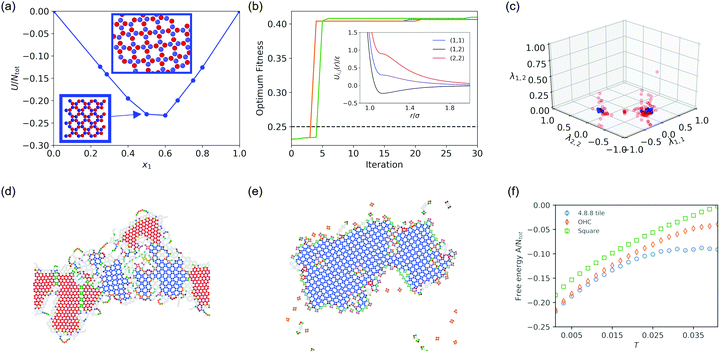Fig. 4 Discovery of the 4.8.8 Archimedean tile. (a) A phase diagram drawn from the stable region at (λ1,1 ≈ −0.295, λ1,2 ≈ 0.226, λ2,2 ≈ −0.894). Species 1 is depicted in blue, species 2 in red. The lower inset with an arrow shows the target used, while the one above it is from MD (T* = 0.007) validating this phase diagram. (b) Evolution of the best fitness found over the course of 3 independent optimization runs. The inset shows the pair potential corresponding to (a). (c) Depiction of the stable region (blue points) where the 4.8.8 tile is found. Other points (not stable) explored by the optimization procedure are depicted in red. Here we used a prior consisting of 125 initial phase diagrams (convex hulls) measured at all combinations of λ1,1 ∈ [−1, −1/2, 0, 1/2, 1], λ1,2 ∈ [0, 1/4, 1/2, 3/4, 1], λ2,2 ∈ [−1, −1/2, 0, 1/2, 1]. (d) Results of MD simulations using individual particles at T* = 0.009. (e) MD of rigid, square tetramers at T* = 0.02. (f) Free energy per particle of three competing polymorphs at finite temperature; the 4.8.8 tile remains the most stable over the entire range.

However, we found that this single component system, containing multiple characteristic length scales in its optimal single-component pair potential, can be exchanged for a binary mixture whose compositional diversity replaces this complexity with a set of simpler interactions. In Fig. 4 we show the inverse design results for this lattice. For validation purposes, we have verified that this lattice can be successfully formed in MD simulations. We emphasize that it can be formed at the same or even higher temperatures used for the single-component case designed via relative entropy optimization (T* = kBT/ε = 0.005).43 For 3 replicates, less than 5 optimization cycles were needed to initially locate stable regions of-space for this lattice, after which only marginal further improvement of the fitness was found. Notably, there are two stable regions found in Fig. 4(c), located symmetrically about the λ1,1 = λ2,2 plane. In this case, the fitness is multimodal, and the structure is stable only over a very narrow region of-space which would almost certainly be missed by a regular grid search.

The stabilization of this tile with simple, isotropic pair potentials is intriguing if one considers the smallest geometric element of the crystal. Another way to view this 4.8.8 Archimedean tile is as an assembly of square tetramers attached to their neighboring squares by their corners [cf.Fig. 4(a)]. Clearly, the 4-fold square symmetry is stable locally within each tetramer, and yet it is not stable globally; if it were, a square lattice, as depicted in red in Fig. 3(a), would result instead. We performed MD simulations using the optimalparameters to investigate further and found that the 4.8.8 tile could be reliably formed across a range of different temperatures; however, it was nearly always accompanied by the formation of some amount of OHC. Fig. 4(d) shows a representative result as identified using the fast neighbor graph analysis algorithm.44 While the final result varies depending on the starting conditions, in the image shown, roughly half of the system has crystallized into each competing polymorph.

To understand this polymorphism thermodynamically, we conducted free energy calculations for the periodic 4.8.8 Archimedean tile, the OHC, and the square lattice using the Einstein molecule approach45,46 as shown in Fig. 4(f). The free energy per particle, A/Ntot, is always lower for the 4.8.8 tile compared to both the OHC and square lattice over the entire temperature range. However, as the ground state is approached, the difference between the OHC and 4.8.8 tile specific free energies converges to approximately 10−3ε/Ntot, in agreement with ground state optimization of their potential energies. This difference is similar to that separating the close-packed HCP and FCC polymorphs,47,48 which are known to crystallize into a polymorphic mixture due to this small free energy difference. Therefore, we attribute the apparent coexistence of the OHC and 4.8.8 tile to kinetic effects which trap some of the system in the less stable OHC phase. Regardless, the free energy calculations validate our inverse design, which accurately predicts the ground state energy rankings to relatively high precision.

As an alternative route to reducing polymorphism, it is possible to imagine an assembly procedure using prefabricated “metaparticles”24,49,50 composed of a set of different monomers irreversibly attached to one another. This multi-stage approach would first require the assembly of these building blocks from individual monomer units, then subsequent mixing of these metaparticles instead of a single stage approach in which the monomers are simply combined. We examined self-assembly using prefabricated rigid dimers and tetramers instead of simple monomers and found that nearly defect-free 4.8.8 Archimedean tiles can be formed by using rigid tetramers, as shown in Fig. 4(e) (cf. ESI). This can be achieved either with rigid square tetramers, or with tetramers which have been minimized at the chosen Hamiltonian resulting in a slightly oblique rhombus. For comparison, we repeated the simulations with the parameters used for stabilizing square lattices (λ1,1 = −1, λ1,2 = 1, λ2,2 = −1) over the same temperature range. Only clusters of squares were observed regardless of the building block used (cf. ESI). Thus, the 4.8.8 Archimedean tile can be formed not only in the ground state but also at finite temperature using the potentials found corresponding to the single-stage assembly directly from monomers, though polymorphism may be reduced by using a multi-stage approach.

## 4 Conclusions

Through a combination of active learning and the calculation of ground state phase diagrams via structure enumeration, this grand canonical inverse design scheme can predict regions of Hamiltonian parameter space that stabilize multicomponent structures, both with respect to polymorphs of the same composition and with respect to phase separation into compositionally distinct structures. We demonstrated this approach's capability to predict isotropic pairwise interactions for binary mixtures that stabilize porous crystals including a Kagome lattice and an Archimedean tile; both of which tend to require anisotropy or more complex pairwise interactions if assembled using single component systems. Our exploration supports the supposition that the ground state phase diagrams of multicomponent mixtures of simple particles contain numerous complex structures which may be stabilized through the correct choice of Hamiltonian variables and mixing ratio. The advantage of using such mixtures is that they may prove more experimentally realizable than their single component counterparts. These results encourage future work on colloidal crystal design using multicomponent systems as they suggest that complex crystals can be feasibly obtained by combining many simple components.

## Conflicts of interest

There are no conflicts to declare.

## Acknowledgements

This work was supported by the U.S. Department of Energy, Office of Basic Energy Science, Division of Material Sciences and Engineering under Award (DE-SC0013979). This research used resources of the National Energy Research Scientific Computing Center, a DOE Office of Science User Facility supported under Contract No. DE-AC02-05CH11231. Use of the high-performance computing capabilities of the Extreme Science and Engineering Discovery Environment (XSEDE), which is supported by the National Science Foundation, Project No. TG-MCB120014, is also gratefully acknowledged. Contribution of the National Institute of Standards and Technology, not subject to US Copyright.

## Notes and references

1. N. Vogel, M. Retsch, C.-A. Fustin, A. del Campo and U. Jonas, Chem. Rev., 2015, 115, 6265–6311 CrossRef CAS PubMed.
2. M. N. O'Brien, M. R. Jones and C. A. Mirkin, Proc. Natl. Acad. Sci. U. S. A., 2016, 113, 11717–11725 CrossRef PubMed.
3. M. A. Boles, M. Engel and D. V. Talapin, Chem. Rev., 2016, 116, 11220–11289 CrossRef CAS PubMed.
4. M. E. Leunissen, C. G. Christova, A.-P. Hynninen, C. P. Royall, A. I. Campbell, A. Imhof, M. Dijkstra, R. van Roij and A. van Blaaderen, Nature, 2005, 437, 235–240 CrossRef CAS PubMed.
5. S. C. Glotzer and M. J. Solomon, Nat. Mater., 2007, 6, 557–562 CrossRef PubMed.
6. S. Sacanna, W. T. M. Irvine, P. M. Chaikin and D. J. Pine, Nature, 2010, 464, 575–578 CrossRef CAS PubMed.
7. D. Klotsa and R. L. Jack, J. Chem. Phys., 2013, 138, 094502 CrossRef PubMed.
8. V. N. Manoharan, Science, 2015, 349, 1253751 CrossRef PubMed.
9. S. Whitelam, 2016, arXiv preprint arXiv:1606.00493.
10. A. W. Long, C. L. Phillips, E. Jankowski and A. L. Ferguson, Soft Matter, 2016, 12, 7119 RSC.
11. X. Zheng, Y. Wang, Y. Wang, D. J. Pine and M. Weck, Chem. Mater., 2016, 28, 3984–3989 CrossRef CAS.
12. M. J. Solomon, Langmuir, 2018, 34, 11205–11219 CrossRef CAS PubMed.
13. P. K. Bommineni, N. R. Varela-Rosales, M. Klement and M. Engel, Phys. Rev. Lett., 2019, 122, 128005 CrossRef CAS PubMed.
14. E. Pretti, H. Zerze, M. Song, Y. Ding, R. Mao and J. Mittal, Sci. Adv., 2019, 5, eaaw5912 CrossRef PubMed.
15. M. Rechtsman, F. Stillinger and S. Torquato, Phys. Rev. E: Stat., Nonlinear, Soft Matter Phys., 2006, 73, 011406 CrossRef PubMed.
16. M. Torikai, J. Chem. Phys., 2015, 142, 144102 CrossRef PubMed.
17. B. A. Lindquist, R. B. Jadrich and T. M. Truskett, J. Chem. Phys., 2016, 145, 111101 CrossRef.
18. R. B. Jadrich, B. A. Lindquist and T. M. Truskett, J. Chem. Phys., 2017, 146, 184103 CrossRef.
19. A. Jain, J. R. Errington and T. M. Truskett, Soft Matter, 2013, 9, 3866–3870 RSC.
20. A. Jain, J. R. Errington and T. M. Truskett, Phys. Rev. X, 2014, 4, 031049 Search PubMed.
21. B. A. Lindquist, R. B. Jadrich, W. D. Piñeros and T. M. Truskett, J. Phys. Chem. B, 2018, 122, 5547–5556 CrossRef CAS PubMed.
22. C. S. Adorf, J. Antonaglia, J. Dshemuchadse and S. C. Glotzer, J. Chem. Phys., 2018, 149, 204102 CrossRef PubMed.
23. B. A. Lindquist, R. B. Jadrich, M. P. Howard and T. M. Truskett, J. Chem. Phys., 2019, 151, 104104 CrossRef PubMed.
24. M. Gruenwald and P. L. Geissler, ACS Nano, 2014, 8, 5891–5897 CrossRef CAS PubMed.
25. K. S. Khalil, A. Sagastegui, Y. Li, M. A. Tahrir, J. E. S. Socolar, B. J. Wiley and B. B. Yellen, Nat. Commun., 2012, 3, 794 CrossRef PubMed.
26. W. M. Jacobs, A. Reinhardt and D. Frenkel, Proc. Natl. Acad. Sci. U. S. A., 2015, 112, 6313–6318 CrossRef CAS PubMed.
27. M. Song, Y. Ding, H. Zerze, M. A. Snyder and J. Mittal, Langmuir, 2018, 34, 991–998 CrossRef CAS PubMed.
28. W. D. Piñeros, B. A. Lindquist and T. M. Truskett, J. Chem. Phys., 2018, 148, 104509 CrossRef PubMed.
29. N. A. Mahynski, E. Pretti, V. K. Shen and J. Mittal, Nat. Commun., 2019, 10, 2028 CrossRef PubMed.
30. H. S. Ashbaugh and H. W. Hatch, J. Am. Chem. Soc., 2008, 130, 9536–9542 CrossRef CAS PubMed.
31. N. A. Mahynski, H. Zerze, H. W. Hatch, V. K. Shen and J. Mittal, Soft Matter, 2017, 13, 5397–5408 RSC.
32. A. Tkachenko, Proc. Natl. Acad. Sci. U. S. A., 2016, 113, 10269–10274 CrossRef CAS PubMed.
33. P. G. Debenedetti, J. Chem. Phys., 1986, 84, 1778 CrossRef CAS.
34. P. G. Debenedetti, Metastable liquids: concepts and principles, Princeton University Press, 1996 Search PubMed.
35. S. Plimpton, J. Comput. Phys., 1995, 117, 1–19 CrossRef CAS.
36. C. E. Rasmussen and K. I. Williams, Gaussian Processes for Machine Learning, MIT Press, 2006 Search PubMed.
37. R. Garnett, M. A. Osborne and P. Hennig, 2013, arXiv preprint arXiv:1310.6740.
38. D. R. Jones, J. Global Optimization, 2001, 21, 345–383 CrossRef.
39. P. Auer, J. Mach. Learn. Res., 2002, 3, 397–422 Search PubMed.
40. N. Srinivas, A. Krause, S. Kakade and M. Seeger, Proceedings of the 27th International Conference on Machine Learning, 2010.
41. Q. Chen, S. C. Bae and S. Granick, Nature, 2011, 469, 381–384 CrossRef CAS PubMed.
42. E. W. Cochran, C. J. Garcia-Cervera and G. H. Frederickson, Macromolecules, 2006, 39, 2449–2451 CrossRef CAS.
43. W. D. Piñeros and T. M. Truskett, J. Chem. Phys., 2017, 146, 144501 CrossRef PubMed.
44. W. F. Reinhart and A. Z. Panagiotopoulos, Soft Matter, 2018, 14, 6083–6089 RSC.
45. C. Vega and E. G. Noya, J. Chem. Phys., 2007, 127, 154113 CrossRef PubMed.
46. E. Pretti and J. Mittal, J. Chem. Phys., 2019, 151, 054105 CrossRef.
47. L. V. Woodcock, Nature, 1997, 385, 141–143 CrossRef CAS.
48. P. G. Bolhuis, D. Frenkel, S.-C. Mau and D. A. Huse, Nature, 1997, 388, 235–236 CrossRef CAS.
49. W. M. Jacobs and D. Frenkel, J. Am. Chem. Soc., 2016, 138, 2457–2467 CrossRef CAS PubMed.
50. M. B. Zanjani, J. C. Crocker and T. Sinno, Soft Matter, 2017, 13, 7098–7105 RSC.

### Footnote

Electronic supplementary information (ESI) available: More details on the fitness function, active learning algorithm, movies, and comparison of phase diagrams to MD simulations. See DOI: 10.1039/c9sm02426c
 This journal is © The Royal Society of Chemistry 2020